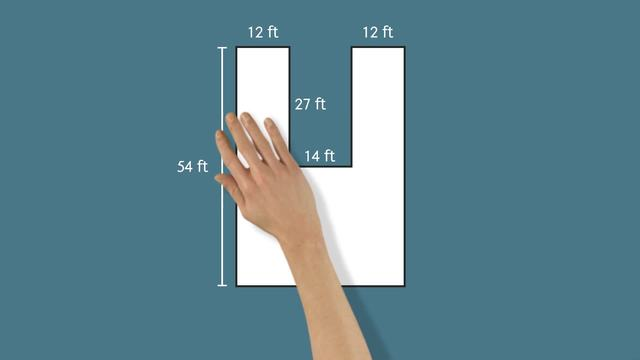# Finding the Area of Polygons Using DecompositionRating

Be the first to give a rating!

The authorsTrentkay

## Basics on the topicFinding the Area of Polygons Using Decomposition

After this lesson, you will be able to find the area of a polygon by decomposing it into smaller shapes.

The lesson begins by teaching you that composite figures can be broken up into smaller regions..often in many different ways! It leads you to learn how to determine the values of missing side lengths in a composite figure. It concludes with an interesting approach to decomposition, using subtraction.

Learn about decomposing polygons by helping Prince Icarus learn to fly!

This video includes key concepts, notation, and vocabulary such as the terms “composite figure” (a shape composed of smaller shapes); “decomposition” (breaking down a shape into smaller regions”; and “area” (the size of a surface or two-dimensional shape).

Before watching this video, you should already be familiar with the formulas for the areas of triangles and rectangles. You should also remember how to use composition and decomposition to find the areas of parallelograms and trapezoids.

After watching this video, you will be prepared to learn how to determine the areas of complex, irregular polygons; and how to determine missing values on a diagram.

Common Core Standard(s) in focus: 6.GA.1 A video intended for math students in the 6th grade Recommended for students who are 11 - 12 years old